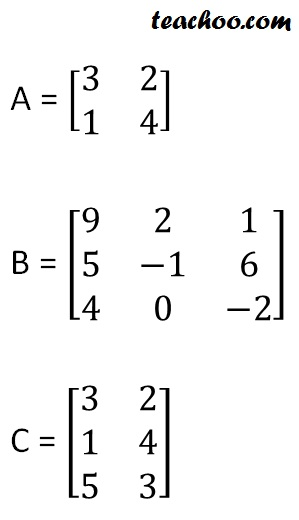Finding determinant of a 2x2 matrix

Chapter 4 Class 12 Determinants
Concept wise

We have studied about matrices before, like,Determinant is a special number calculated from square matrix

So, determinant of matrix A & B is possible

But

Determinant’s of matrix C is not possible

Because matrix C is not a square matrix

For matrix A,

We denote determinant as

det A

or

det (A)

or

|A|

Get live Maths 1-on-1 Classs - Class 6 to 12

### Transcript

A = [3 2 1 4] B = [9 2 1 5 -1 6 4 0 -2] C = [3 2 1 4]